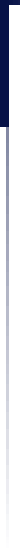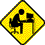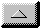Ask Dr. SETI: Departments     Membership Services    General Info    Financial Info    Activities    Awards    Coordinators    Director's Info    Members' Info    Policies    Forms  Publications    Official Publications    Director's Publications    Ask Dr. SETI ®    Fiction    Non-Fiction    Reviews    Reading Lists  Technical Support    Systems    Antennas    Amplifiers    Receivers    Accessories    Hardware    Software  Press Relations    Fact Sheets    Local Contacts    Editorials    Press Releases    Photo Gallery    Newsletters    Internet SvcsChapter 1: Astrophysics Characteristic Impedance of Free Space Dear Dr. SETI: I am a little confused about the value for free space characteristic imepdance. The SETI League's literature sets the impedance of free space at 120 pi, or about 377 ohms. In the engineering literature, I find: 376.730313462 ohms (not exactly 120 pi). Why the disprepancy? Wim, Netherlands The Doctor Responds: Although one might rightly argue that the discrepancy is neglibible, you're wise to question the exact value, because this is the impedance to which one end (the radiating end) of our antennas must be matched. The characteristic (or surge) impedance of any transmission medium is the impedance we would encounter if looking into an infinitely long segment of that medium. Since we can consider free space to be, for all practical purposes, infinite in extent, any antenna will appear to be terminated in this calculable impedance. One way to define impedance is as the ratio of potential to kinetic energy in a dynamic system. Electromotive force is a manifestation of electrical potential energy (we even call voltage 'electrical potential'), and current is the rate of flow of charged particles, which is a manifestation of kinetic energy. Thus, we describe impedance as: Z = e / i (You probably know of this relationship as as Ohm's Law.) For propagation of electromagnetic energy, the impedance has to be somewhere between zero ohms and infinity. This is because power is the product of potential and current, the potential across the short would be zero (yielding a zero product), and the current through an open is zero (also resulting in a zero product). But, how do we find where the actual impedance of free space falls within this rather wide range? Consider that an electrical potential gives rise to an electrostatic field, the intensity of which varies inversely with the square root of the permittivity of the medium in which it propagates. Similarly, an electrical current results in the creation of a magnetic field, the intensity of which is inversely proportional to the square root of the permeability of the medium in which it propagates. So, if we know the permittivity and permeability of free space, we can determine the impedance to which an electromagnetic wave propagating in free space is subject. The relationship is: Zo = sqrt (muo / epsilono) where epsilon represents permittivity (in Farads per meter), mu is permeability (in Henries per meter), Z means impedance, and the subscript o indicates free space. The relevant dimensions for Zo would be the square root of {(H/m)/(F/m)}, which equates to sqrt{H/F), which reduces to ohms. All we need now is to know the values of permittivity and permeability for free space, and we can solve for characteristic impedance. Here are a couple of close approximations: epsilono ~ [1 / (36 pi)] x 10-9 F/m, and muo ~ (4 pi) x 10-7 H/m Solving for free space characteristic impedance, we insert the above characteristics into the impedance equation: Zo = sqrt (muo / epsilono) (H / F)1/2 Zo = sqrt { (4 pi) x 10-7 / [1 / (36 pi)] x 10-9 } ohms Zo = sqrt { 36 x 4 x pi2 x 102 } ohms Zo = { 6 x 2 x pi x 10 } ohms Zo = { 120 x pi } ohms Of course, this value is only as accurate as our approximations of free space permittivity and permeability. It turns out the values cited are not precise, but are accurate to within a few hundredths of a percent. Which is why our estimate of 120 pi ohms is similarly accurate to only a few hundredths of a percent. But, how close did we come, in practical terms? If you match an antenna to an impedance of 120 pi ohms, instead of the more precise value you cited in your question, an impedance mismatch will arise, resulting in a voltage standing wave ratio of 1.00069 : 1. Since a VSWR of 1 : 1 represents a perfect impedance match, I think you will agree that the discrepancy is negligible. As an aside, I should point out that the values of permittivity and permeability of free space also allow us to calculate the speed of light. Using the approximations above yields the generally accepted value of c = 3 x 108 m/s. Using more precise values for mu and epsilon, we get a more precise value of c = 2.998 x 108 m/s. Like the impedance discrepancy, the difference is, for all practical purposes, nonexistent.email theWebmaster | Home | General | Memb Svcs | Publications | Press | Technical | Internet | Index | entire website copyright © The SETI League, Inc.; Maintained by Microcomm this page last updated 20 January 2007Top of Page